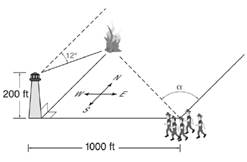Chapter 11.3, Problem 45EElementary Geometry For College St...

7th Edition
Alexander + 2 others
ISBN: 9781337614085

Solutions

Chapter
SectionElementary Geometry For College St...

7th Edition
Alexander + 2 others
ISBN: 9781337614085
Textbook Problem

In Exercises 39 to 45, angle measures should be given to the nearest degree; distance should be given to the nearest tenth of a unit.From atop a 200 -ft lookout tower, a fire is spotted due north through an angle of depression of 12 ∘ . Firefighters located 1000 ft due east of the tower must work their way through heavy foliage to the fire. By their compasses, through what angle (measured from the north toward the west) must the firefighters travel?To determine

To calculate:

The angle measured from the north toward the west must the firefighters travel.

Explanation

Given:

From a top 200ft lookout tower, a fire is spotted due to north through an angle of depression of 12 degree. Firefighters located heavy foliage to the fire.

Calculation:

Let h be the height of the tower, d be the distance from the fire to the tower and β be the angle of depression.

tanβ=hdtan12=200dd=200tan12940.92602

Let us assume that d be the distance from firefight to the tower

Still sussing out bartleby?

Check out a sample textbook solution.

See a sample solution

The Solution to Your Study Problems

Bartleby provides explanations to thousands of textbook problems written by our experts, many with advanced degrees!

Get Started

For the following scores, find the value of each expression: X 3 2 4 2 a. X b. (X)2 c. X 2 d. (X 2)

Essentials of Statistics for The Behavioral Sciences (MindTap Course List)

f(x) = (x + 3)3/2

Applied Calculus for the Managerial, Life, and Social Sciences: A Brief Approach

For

Study Guide for Stewart's Multivariable Calculus, 8th

Sometimes, Always, or Never: If 0 ≤ an ≤ bn for all n and diverges, then converges.

Study Guide for Stewart's Single Variable Calculus: Early Transcendentals, 8th